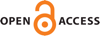Download this articleFor screen For printingRecent IssuesThe Journal About the Journal Editorial Board Editorial Interests Editorial Procedure Subscriptions Submission Guidelines Submission Page Policies for Authors Ethics Statement ISSN (electronic): 1364-0380 ISSN (print): 1465-3060 Author Index To Appear Other MSP JournalsAlgebraic Spivak's theorem and applications

### Toni Annala

Geometry & Topology 27 (2023) 351–396##### Abstract

We prove an analogue of Lowrey and Schürg’s algebraic Spivak’s theorem when working over a base ring $A$ that is either a field or a nice enough discrete valuation ring, and after inverting the residual characteristic exponent $e$ in the coefficients. By this result algebraic bordism groups of quasiprojective derived $A$–schemes can be generated by classical cycles, leading to vanishing results for low-degree $e$–inverted bordism classes, as well as to the classification of quasismooth projective $A$–schemes of low virtual dimension up to $e$–inverted cobordism. As another application, we prove that $e$–inverted bordism classes can be extended from an open subset, leading to the proof of homotopy invariance of $e$–inverted bordism groups for quasiprojective derived $A$–schemes.

##### Keywords
algebraic bordism, algebraic cobordism, derived algebraic geometry
##### Mathematical Subject Classification
Primary: 14F43
Secondary: 14C99, 14J99
##### Publication